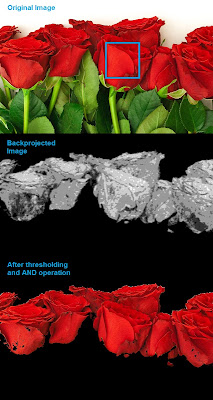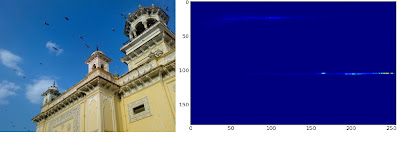close

# OpenCV-Python

### OpenCV Python Tutorials

opencvpython.blogspot.com

## Histograms - 4 : Backprojection

Hi friends,

Today, we will look into histogram back-projection. It was proposed by Michael J. Swain , Dana H. Ballard in their paper "Indexing via color histograms".

Well, what is it actually in simple words? It is used for image segmentation or finding objects of interest in an image. In simple words, it creates an image of the same size (but single channel) as that of our input image, where each pixel corresponds to the probability of that pixel belonging to our object. So in short, the output image will have our object of interest in white and remaining part in black. Well, that is an intuitive explanation.

(In this article, I would like to use a beautiful image of a bunch of rose flowers. And the image credit goes to "mi9.com". You can get the image from this link : http://imgs.mi9.com/uploads/flower/4649/rose-flower-wallpaper-free_1920x1080_83181.jpg)

How do we do it ? We create a histogram of an image containing our object of interest (in our case, the rose flower, leaving leaves and background). The object should fill the image as far as possible for better results. And a color histogram is preferred over grayscale histogram, because color of the object is more better way to define the object than its grayscale intensity. ( A red rose flower and its green leaves may have same intensity in grayscale images, but easily distinguishable in color image). We then "back-project" this histogram over our test image where we need to find the object, ie in other words, we calculate the probability of every pixel belonging to rose flower and show it. The resulting output on proper thresholding gives us the rose flower alone.

So let's see how it is done.

Algorithm :

1 - First we need to calculate the color histogram of both the object we need to find (let it be 'M') and the image where we are going to search (let it be 'I').

`import cv2import numpy as npfrom matplotlib import pyplot as plt#roi is the object or region of object we need to findroi = cv2.imread('rose_red.png')hsv = cv2.cvtColor(roi,cv2.COLOR_BGR2HSV)#target is the image we search intarget = cv2.imread('rose.png')hsvt = cv2.cvtColor(target,cv2.COLOR_BGR2HSV)# Find the histograms. I used calcHist. It can be done with np.histogram2d alsoM = cv2.calcHist([hsv],[0, 1], None, [180, 256], [0, 180, 0, 256] )I = cv2.calcHist([hsvt],[0, 1], None, [180, 256], [0, 180, 0, 256] )`

2 - Find the ratio R = M/I

`R = M/(I+1)`

3 - Now backproject R, ie use R as palette and create a new image with every pixel as its corresponding probability of being target. ie B(x,y) = R[h(x,y),s(x,y)] where h is hue and s is saturation of the pixel at (x,y). After that apply the condition B(x,y) = min[B(x,y), 1].

`h,s,v = cv2.split(hsvt)B = R[h.ravel(),s.ravel()]B = np.minimum(B,1)B = B.reshape(hsvt.shape[:2])`

4 - Now apply a convolution with a circular disc, B = D * B, where D is the disc kernel.

`disc = cv2.getStructuringElement(cv2.MORPH_ELLIPSE,(5,5))cv2.filter2D(B,-1,disc,B)B = np.uint8(B)cv2.normalize(B,B,0,255,cv2.NORM_MINMAX)`

5 - Now the location of maximum intensity gives us the location of object. If we are expecting a region in the image, thresholding for a suitable value gives a nice result.

`ret,thresh = cv2.threshold(B,50,255,0)`

Below is one example I worked with. I used the region inside blue rectangle as sample object and I wanted to extract all the red roses. See, ROI is filled with red color only :Histogram Backprojection

Backprojection in OpenCV

OpenCV provides an inbuilt function cv2.calcBackProject(). Its parameters are almost same as the cv2.calcHist() function. One of its parameter is histogram which is histogram of the object and we have to find it. Also, the object histogram should be normalized before passing on to the backproject function. It returns the probability image. Then we convolve the image with a disc kernel and apply threshold. Below is my code and output :

`import cv2import numpy as nproi = cv2.imread('rose_green.png')hsv = cv2.cvtColor(img,cv2.COLOR_BGR2HSV)target = cv2.imread('rose.png')hsvt = cv2.cvtColor(target,cv2.COLOR_BGR2HSV)# calculating object histogramroihist = cv2.calcHist([hsv],[0, 1], None, [180, 256], [0, 180, 0, 256] )# normalize histogram and apply backprojectioncv2.normalize(roihist,roihist,0,255,cv2.NORM_MINMAX)dst = cv2.calcBackProject([hsvt],[0,1],roihist,[0,180,0,256],1)# Now convolute with circular discdisc = cv2.getStructuringElement(cv2.MORPH_ELLIPSE,(5,5))cv2.filter2D(dst,-1,disc,dst)# threshold and binary ANDret,thresh = cv2.threshold(dst,50,255,0)thresh = cv2.merge((thresh,thresh,thresh))res = cv2.bitwise_and(target,thresh)res = np.vstack((target,thresh,res))cv2.imwrite('res.jpg',res)`

Below is the output. Here ROI is not just flower, but some green part is also included. Still output is good. On close analysis of the center image, you can see the leaf parts slightly which will be removed on threshold :Histogram Backprojection in OpenCV

Summary

So we have looked on what is Histogram backprojection, how to calculate it, how it is useful in object detection etc. It is also used in more advanced object tracking methods like camshift. We will do that later.

Regards,
Abid Rahman K.

References :

1 - "Indexing via color histograms", Swain, Michael J. , Third international conference on computer vision,1990.
2 - http://www.codeproject.com/Articles/35895/Computer-Vision-Applications-with-C-Part-II
3 - http://theiszm.wordpress.com/tag/backprojection/

## Histograms - 3 : 2D Histograms

Hi friends,

In the first article, we calculated and plotted one-dimensional histogram. It is called one-dimensional because we are taking only one feature into our consideration, ie grayscale intensity value of the pixel. But in two-dimensional histograms, you consider two features. Normally it is used for finding color histograms where two features are Hue & Saturation values of every pixel.

There is a python sample in the official samples already for finding color histograms. We will try to understand how to create such a color histogram, and it will be useful in understanding further topics like Histogram Back-Projection.

2D Histogram in OpenCV

It is quite simple and calculated using the same function, cv2.calcHist(). For color histogram, we need to convert the image from BGR to HSV. (Remember, for 1D histogram, we converted from BGR to Grayscale). While calling calcHist(), parameters are :

channels = [0,1] # because we need to process both H and S plane.
bins = [180,256] # 180 for H plane and 256 for S plane
range = [0,180,0,256] # Hue value lies between 0 and 180 & Saturation lies between 0 and 256

`import cv2import numpy as npimg = cv2.imread('home.jpg')hsv = cv2.cvtColor(img,cv2.COLOR_BGR2HSV)hist = cv2.calcHist( [hsv], [0, 1], None, [180, 256], [0, 180, 0, 256] )`

That's it.

2D Histogram in Numpy

Numpy also provides a specific function for this : np.histogram2d(). (Remember, for 1D histogram we used np.histogram() ).

`import cv2import numpy as npfrom matplotlib import pyplot as pltimg = cv2.imread('home.jpg')hsv = cv2.cvtColor(img,cv2.COLOR_BGR2HSV)hist, xbins, ybins = np.histogram2d(h.ravel(),s.ravel(),[180,256],[[0,180],[0,256]])`

First argument is H plane, second one is the S plane, third is number of bins for each and fourth is their range.

Now we can check how to plot this color histogram

Plotting 2D Histogram

Method - 1 : Using cv2.imshow()
The result we get is a two dimensional array of size 180x256. So we can show them as we do normally, using cv2.imshow() function. It will be a grayscale image and it won't give much idea what colors are there, unless you know the Hue values of different colors.

Method - 2 : Using matplotlib
We can use matplotlib.pyplot.imshow() function to plot 2D histogram with different color maps. It gives us much more better idea about the different pixel density. But this also, doesn't gives us idea what color is there on a first look, unless you know the Hue values of different colors. Still I prefer this method. It is simple and better.

NB : While using this function, remember, interpolation flag should be 'nearest' for better results.

`import cv2import numpy as npfrom matplotlib import pyplot as pltimg = cv2.imread('home.jpg')hsv = cv2.cvtColor(img,cv2.COLOR_BGR2HSV)hist = cv2.calcHist( [hsv], [0, 1], None, [180, 256], [0, 180, 0, 256] )plt.imshow(hist,interpolation = 'nearest')plt.show()`

Below is the input image and its color histogram plot. X axis shows S values and Y axis shows Hue.2D Histogram in matplotlib with 'heat' color map

In histogram, you can see some high values near H = 100 and S = 200. It corresponds to blue of sky. Similarly another peak can be seen near H = 25 and S = 100. It corresponds to yellow of the palace. You can verify it with any image editing tools like GIMP.

Method 3 : OpenCV sample style !!
There is a sample code for color_histogram in OpenCV-Python2 samples. If you run the code, you can see the histogram shows even the corresponding color. Or simply it outputs a color coded histogram. Its result is very good (although you need to add extra bunch of lines).

In that code, the author created a color map in HSV. Then converted it into BGR. The resulting histogram image is multiplied with this color map. He also uses some preprocessing steps to remove small isolated pixels, resulting in a good histogram.

I leave it to the readers to run the code, analyze it and have your own hack arounds. Below is the output of that code for the same image as above:OpenCV-Python sample color_histogram.py output

You can clearly see in the histogram what colors are present, blue is there, yellow is there, and some white due to chessboard(it is part of that sample code) is there. Nice !!!

Summary :

So we have looked into what is 2D histogram, functions available in OpenCV and Numpy, how to plot it etc.

So this is it for today !!!

Regards,
Abid Rahman K.

## Report "OpenCV-Python"

Are you sure you want to report this post for ?

Cancel
×